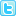# Abstract

## A unified study of fractional kinetic equation and thermonuclear functions

The paper discusses the solution of a simple kinetic equation of the type used for the computation of the change of the chemical composition in stars like the sun. Starting from the standard form of the kinetic equation it is generalized to a fractional kinetic equation and its solutions in terms of H -functions are obtained. The role of thermonuclear functions, which are also represented in terms of H- and H - functions. In such a fractional kinetic equation is emphasized. Results contained in this paper are related to recent investigations of possible astrophysical solutions of the solar neutrino problem.

Author(s): Yashwant Singh and Naseem A. Khan

Abstract | PDF

Share thisOsaka, Japan
• ### 20th Annual Meet on Pharmaceutical Sciences

Bangkok, Thailand
###### Abstracted/Indexed in
• Chemical Abstracts Service (CAS)
• Index Copernicus